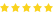Display: List Grid
Sort By:
Show:

### Body Maxx 10 Kg PVC Weight Plates, 5 and 3 ft Rod, 2 D. Rods Home Gym Equipment Dumbbell Set.

INR 3,060.00 INR 1,120.00

Body Maxx 10 Kg of PVC weight (2kg X 2 = 4kg + 3kg X 2 =6kg ) 1x5 feet plain rod (Shoulder or.....

### Body Maxx 100 Kg PVC Weight Plates, 5 and 3 ft Rod, 2 D. Rods Home Gym Equipment Dumbbell Set.

INR 9,150.00 INR 4,499.00Body Maxx 100 Kg of PVC weight (10kg X 4 = 20kg + 5kg X 4 =20kg + 2.5kg X 8 = 20kg + 2kg x 4 .....

### Body Maxx 15 Kg PVC Weight Plates, 5 and 3 ft Rod, 2 D. Rods Home Gym Equipment Dumbbell Set.

INR 3,390.00 INR 1,279.00

Body Maxx 15 Kg of PVC weight (5kg X 2 = 10kg + 2.5kg X 2 =5kg ) 1x5 feet plain rod (Shoulder.....

### Body Maxx 20 Kg PVC Weight Plates, 5 and 3 ft Rod, 2 D. Rods Home Gym Equipment Dumbbell Set.

INR 3,730.00 INR 1,449.00

Body Maxx 20 Kg of PVC weight (2kg X 4 = 8kg + 3kg X 4 =12kg ) 1x5 feet plain rod (Shoulder o.....

### Body Maxx 25 Kg PVC Weight Plates, 5 and 3 ft Rod, 2 D. Rods Home Gym Equipment Dumbbell Set.

INR 4,075.00 INR 1,610.00

Body Maxx 25 Kg of PVC weight (10kg X 2 = 20kg + 2.5kg X 2 =5kg ) 1x5 feet plain rod (Shoulde.....

### Body Maxx 30 Kg PVC Weight Plates, 5 and 3 ft Rod, 2 D. Rods Home Gym Equipment Dumbbell Set.

INR 4,405.00 INR 1,770.00Body Maxx 30 Kg of PVC weight (10kg X 2 = 20kg + 2.5kg X 4 =10kg ) 1x5 feet plain rod (Should.....

### Body Maxx 35 Kg PVC Weight Plates, 5 and 3 ft Rod, 2 D. Rods Home Gym Equipment Dumbbell Set.

INR 4,740.00 INR 1,929.00

Body Maxx 35 Kg of PVC weight (10kg X 2 = 20kg + 5kg X 2 =10kg + 2.5kg X 2 = 5kg ) 1x5 feet p.....

### Body Maxx 40 Kg PVC Weight Plates, 5 and 3 ft Rod, 2 D. Rods Home Gym Equipment Dumbbell Set.

INR 5,090.00 INR 2,099.00

Body Maxx 40 Kg of PVC weight (10kg X 2 = 20kg + 3kg X 4 =12kg + 2kg X 4 = 8kg ) 1x5 feet pla.....

### Body Maxx 45 Kg PVC Weight Plates, 5 and 3 ft Rod, 2 D. Rods Home Gym Equipment Dumbbell Set.

INR 5,420.00 INR 2,259.00

Body Maxx 45 Kg of PVC weight (10kg X 2 = 20kg + 5kg X 4 =20kg + 2.5kg X 2 = 5kg ) 1x5 feet p.....

### Body Maxx 50 Kg PVC Weight Plates, 5 and 3 ft Rod, 2 D. Rods Home Gym Equipment Dumbbell Set.

INR 5,780.00 INR 2,599.00Body Maxx 50 Kg of PVC weight (10kg X 2 = 20kg + 5kg X 4 =20kg + 2.5kg X 4 = 10kg ) 1x5 feet .....

### Body Maxx 60 Kg PVC Weight Plates, 5 and 3 ft Rod, 2 D. Rods Home Gym Equipment Dumbbell Set.

INR 6,445.00 INR 2,749.00

Body Maxx 60 Kg of PVC weight (10kg X 2 = 20kg + 5kg X 4 =20kg + 2.5kg X 8 = 20kg ) 1x5 feet .....

### Body Maxx 70 Kg PVC Weight Plates, 5 and 3 ft Rod, 2 D. Rods Home Gym Equipment Dumbbell Set.

INR 6,999.00 INR 3,070.00

Body Maxx 70 Kg of PVC weight (10kg X 2 = 20kg + 5kg X 4 =20kg + 2.5kg X 4 = 10kg + 2kg x 4 =.....

### Body Maxx 80 Kg PVC Weight Plates, 5 and 3 ft Rod, 2 D. Rods Home Gym Equipment Dumbbell Set.

INR 7,799.00 INR 3,399.00

Body Maxx 80 Kg of PVC weight (10kg X 2 = 20kg + 5kg X 6 =30kg + 2.5kg X 4 = 10kg + 2kg x 4 =.....

### Body Tech 12Kg-Combo With 15 Inches Dumbells Rod

INR 1,399.00 INR 440.00

Each dumbbell rod can accommodate maximum of two weight plates on either side . Possible combinat.....

### Body Tech 15Kg-Combo With 15 Inches Dumbells Rod

INR 1,560.00 INR 520.00

Each dumbbell rod can accommodate maximum of two weight plates on either side . Possible combinat.....